## February 09, 2010

### How powerful is a physics professor, anyway?Over at Dot Physics, Rhett has been explaining the inaccurate calculation of power on a TV show that I have never heard of or had the chance to see. He posted a video of himself pulling the family minivan with the family inside. He even posted a video of his 6-year old pulling the vehicle!

I assume that in a future post, he'll be giving the right way to calculate the power, but I couldn't resist trying out video analysis in Logger Pro with his video.

I analyzed the first 3.5 seconds after the van starts to move by clicking on the same point on the van every few frames. I used the wheel base of the 2007 Mazda 5 as a guess for the scale calibration.I was pretty surprised that on my first attempt, the position-time data had a nice parabolic shape. I could have probably spent a little more time making sure that I always clicked on the exact same spot on the van, but for quick and dirty analysis, I'm pretty happy. Logger Pro defaults to motion to the left as having negative velocity. I didn't really try to reset this, as I only care about the magnitude of the rate of velocity change (i.e. the acceleration).

The acceleration is the slope of the velocity-time graph, so let's look at that: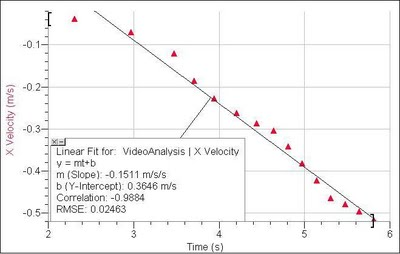Okay, so Rhett either wasn't applying a constant force over the 3.5 seconds, or my measurement uncertainties are starting to show up. Either way, the average acceleration can be estimated by looking at the slope of the best fit line. Logger Pro says that the average acceleration was 0.15 m/s2.

So, using Rhett's free body diagram, we can apply Newton's second law: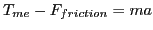The friction force in this case is rolling friction, so we need a coefficient of rolling resistance. The friction force is equal to the coefficient of rolling resistance times the normal force. The normal force (on the level ground) is just the mass of the vehicle multiplied by the acceleration due to gravity.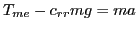Rearrange to solve for the applied force: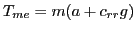I googled around a bit and found that a typical auto on concrete has a coefficient of rolling resistance between 0.010 and 0.015, so I ballparked it at 0.012. Rhett gave the mass of the car in pounds, so I converted to kilograms.

Finally: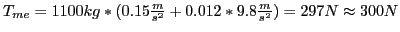I didn't carry out the video analysis the whole way through the entire movie. The van moves for about 9 seconds, and I know its acceleration was not constant, since the brakes were applied at the end. However, I'm sure that our physics professor could sustain the constant 300 N applied force if he wanted to all the way through the 5 yards (strange units they use in LA; we'll call it 4.57 meters). At the constant acceleration we measured, it should take him 7.8 seconds to pull the van that far.

So the work done by our professor is equal to the force applied (300 N) multiplied by the distance through which the force acted (4.57 m). His power output during the pull is the work done divided by the time:

300 N * 4.57 m / 7.8 s = 176 W

That's a mighty bright light bulb, but it's just under twice the power radiated (on average) by a human that is just standing around doing nothing.

I know he was pulling at an angle, so his applied force is larger by the cosine of the angle, which I guesstimated to by 15 degrees, so make the applied force 310 N and the power 182 W if you want to be nitpicky about it...